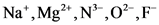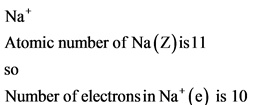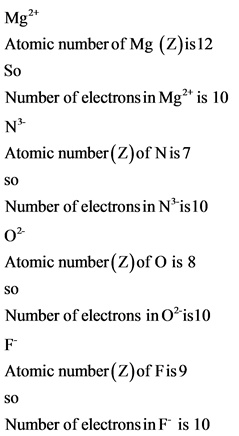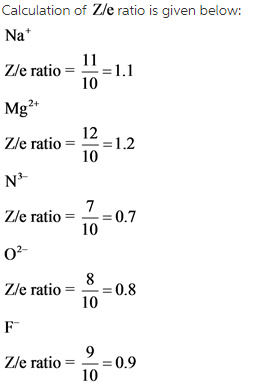# Rank these ions according to ionic radius from largest to smallest:

Rank these ions according to ionic radius from largest to smallest:
Na+ Mg2+ N3- O2- F-

Concepts and reason
Ions are formed when neutral atom accepts or donates the electrons. Ionic radius may be smaller or larger depending on the charge present in it. Ionic radius is the distance between the nucleus and valence shell of an ion. For ions with the isoelectronic series (same number of electrons and different number of protons), the order of ionic radii can be identified by finding the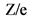ratio.

Fundamentals
Isoelectronic series: The ions having the equal number of electrons, the size depends onratio. The term “Z” is atomic number and “e” is number of electrons.
oAs theratio increases, the ionic radius decreases due to a greater nuclear force on the valence shell.
oAs theratio decreases, the ionic radius increases due to a lesser nuclear force on the valence shell.